# Fisher Price Index

A consumer price index used to measure the price level of goods and services over a given period

## What is the Fisher Price Index?

The Fisher Price Index, also called the Fisher’s Ideal Price Index, is a consumer price index (CPI) used to measure the price level of goods and services over a given period. The Fisher Price Index is a geometric average of the Laspeyres Price Index and the Paasche Price Index. It is deemed the “ideal” price index as it corrects the positive price bias in the Laspeyres Price Index and the negative price bias in the Paasche Price Index.### Understanding the Index

Similar to other consumer price indices, the Fisher Price Index is used to measure the price level and cost of living in an economy and to calculate inflation. The index corrects for the upward bias of the Laspeyres Price Index and the downward bias of the Paasche Price Index by taking the geometric average of the two weighted indices.

### Formula for the Fisher Price Index

The Fisher Price Index is the geometric average of the Laspeyres and Paasche Price indices, and the formula is rendered as: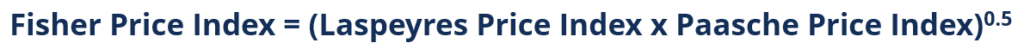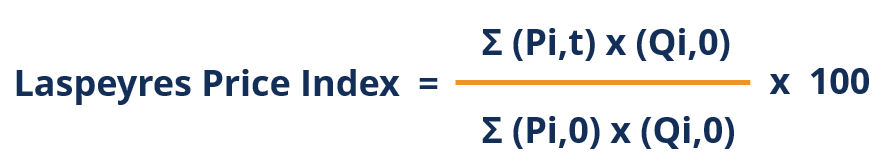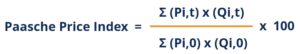Where:

• Pi,t is the price of the individual item at the observation period
• Pi,0 is the price of the individual item at the base period
• Qi,t is the quantity of the individual item at the observation period
• Qi,0 is the quantity of the individual item at the base period

### How to Calculate the Fisher Price Index

The index requires a fair amount of computations. The steps taken to calculate the Index should be as follows:

Step 1: Calculate the Laspeyres Price Index for each period. Remember that the Laspeyres Price Index uses observation price and base quantities in the numerator and base price and base quantities in the denominator.

Step 2: Calculate the Paasche Price Index for each period. Remember that the Paasche Price Index uses observation price and observation quantities in the numerator and base price and base quantities in the denominator.

Step 3: Take the geometric average of the Laspeyres and Paasche Price Index in each period to determine the Fisher Price Index for the corresponding period.

### Practical Example

The following information regarding the change in price and quantities of each individual good in a hypothetical economy is provided. Determine the Fisher Price Index for Year 0, Year 1, and Year 2, using Year 0 as the base year.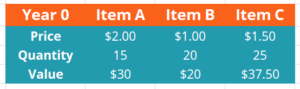Using Year 0 as the base year, all of the price indexes for that year should be 100. For completeness, the calculations are shown below: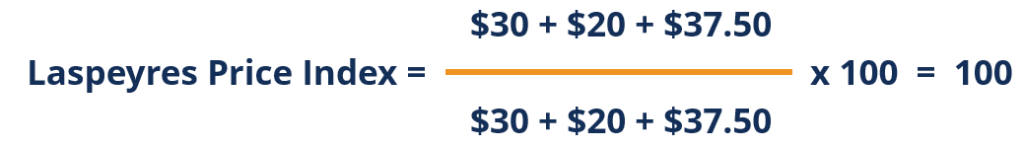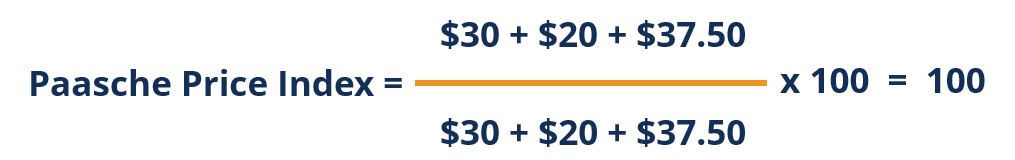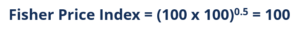#### Year 1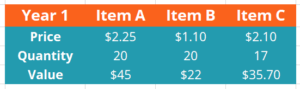Recall that the Laspeyres Price Index uses observation price and base quantities in the numerator and base price and base quantities in the denominator: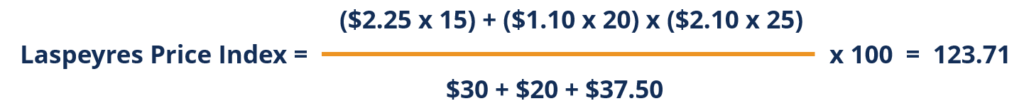Recall that the Paasche Price Index uses observation price and observation quantities in the numerator and base price and base quantities in the denominator: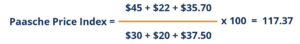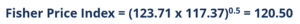#### Year 2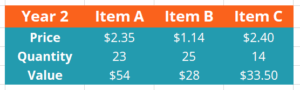Recall that the Laspeyres Price Index uses observation price and base quantities in the numerator and base price and base quantities in the denominator: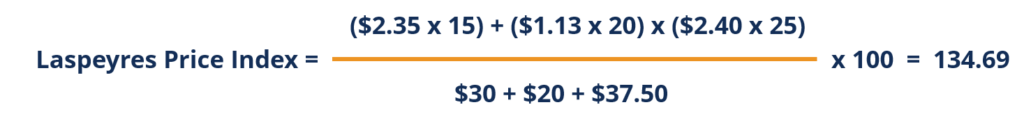Recall that the Paasche Price Index uses observation price and observation quantities in the numerator and base price and base quantities in the denominator: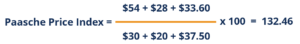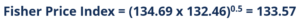Below is a summarized table of the Laspeyres, Paasche, and Fisher Price Index for each year: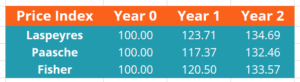As you can see, the Fisher Index number lies between the Laspeyres and Paasche Price Index numbers!

### More Resources

CFI is the official provider of the global Financial Modeling & Valuation Analyst (FMVA)™ certification program, designed to help anyone become a world-class financial analyst. To keep advancing your career, the additional CFI resources below will be useful:

• Disinflation
• Normal Goods
• Phillips Curve
• Pigou Effect

### Financial Analyst Certification

Become a certified Financial Modeling and Valuation Analyst (FMVA)® by completing CFI’s online financial modeling classes!